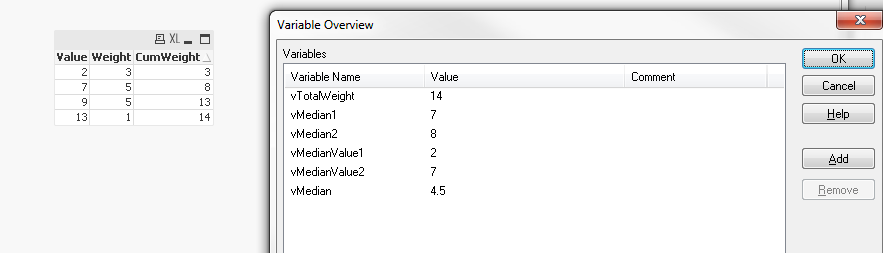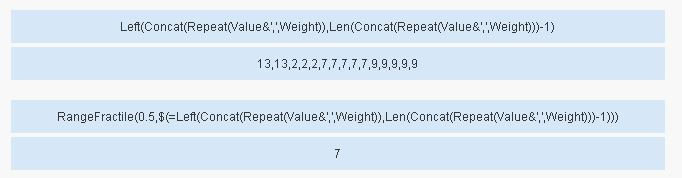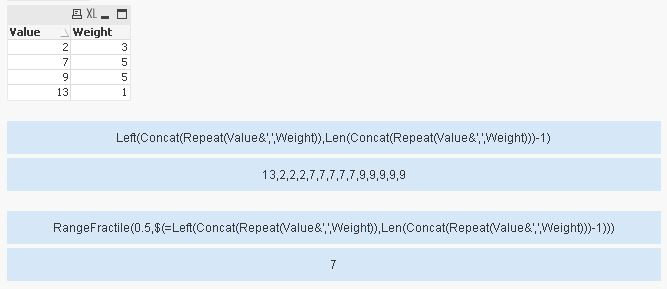# QlikView App Development

Discussion Board for collaboration related to QlikView App Development.

Contributor

## Weighted median

Can anyone suggest the best way to calculated a weighted median in QlikView

The data i have is in the following format.

Value, Weight

2, 3

7, 5

9, 5

13, 2

The weighted median is 7 as illustrated below.

2 2 2 7 7 7 7 7 9 9 9 9 9 13 13

Can anyone think of the best way to calculated this in QlikView?

Thanks

Matt

1 Solution

Accepted SolutionsMVP

## Re: Weighted median

Hi,

maybe like:

```Aggr(If(Rangesum(Above(Weight,0,RowNo()))/Sum(TOTAL Weight)>=0.5 and Rangesum(Above(Weight,1,RowNo()-1))/Sum(TOTAL Weight)<0.5,Value),Value)
```hope this helps as well

regards

Marco

23 RepliesPartner

## Re: Weighted median

Hi Matt,

you need to use Median function.

• median ([{set_expression}] [ distinct ] [ total [<fld {,fld}>] ] expression )

Median([Value])

Regards,

Ely

Contributor

## Re: Weighted median

Thanks Ely,

But that won't take the weight into account, the weighted median is 7, just doing median of Value gives me 8

Any ideas?

Cheers

MattPartner

## Re: Weighted median

Hi Matt,

I have created a new QlikView document with your list of value, 15 not distinct values in field.

Check  return number of this function Count([Value])

Regards,

Ely

Contributor

## Re: Weighted median

Thank Ely - I have the same here with the expanded list, I was wondering if there if there was a way of using the two fields in my data

Value, Weight

2, 3

7, 5

9, 5

13, 2

to come up with the same answer in one expression. Or whether (as you and I have done) I need to first expand the data?

If the only way to do this is to expand the data into the list of 15 values can you think of a quick way of doing this in a script without looping through the list with peek, which would be very slow with high data volumes. Also the weight my not necessarily be a whole number in every scenario that we may want to use this?

MattMVP

## Re: Weighted median

Try this script:

Table:

Value, Weight

2, 3

7, 5

13, 2

9, 5

];

NewTable:

RangeSum(Weight, Peek('CumWeight')) as CumWeight

Resident Table

Order By Value;

LET vTotalWeight = Peek('CumWeight');

LET vMedian1 = If(Odd(\$(vTotalWeight)), Ceil(\$(vTotalWeight)/2), \$(vTotalWeight)/2);

LET vMedian2 = If(Odd(\$(vTotalWeight)), Ceil(\$(vTotalWeight)/2), (\$(vTotalWeight)/2 + 1));

Temp1:

NoConcatenate

Weight

Resident NewTable

Where CumWeight <= \$(vMedian1);

LET vMedianValue1 = Peek('Value1');

Temp2:

NoConcatenate

Weight

Resident NewTable

Where CumWeight <= \$(vMedian2);

LET vMedianValue2 = Peek('Value2');

DROP Tables Temp1, Temp2, Table;

LET vMedian = (\$(vMedianValue1) + \$(vMedianValue2))/2;

The median value is saved in the variable vMedian.

Variable Overview:When the data is changed so that the sum of weight is 14 and the median will be the average of 7th observation and 8th observation you get the following result ->MVP

## Re: Weighted median

Hi,

one solution could be:

```RangeFractile(0.5,\$(=Left(Concat(Repeat(Value&',',Weight)),Len(Concat(Repeat(Value&',',Weight)))-1)))
```hope this helps

regards

MarcoMVP

## Re: Weighted median

Hey Marco this is an amazing way to handle this. I have never used the repeat function before and was thinking of something like this in script. Is there a repeat equivalent of something in script?

Also, I am unable to open your attached qvw because I am using the PE, but can you check what is the median if this the data:

Table:

Value, Weight

2, 3

7, 5

13, 1

9, 5

];

I would expect it to be 4.5

Thanks,

SunnyMVP

## Re: Weighted median

Hi Sunny,

you can use repeat() in the script as well.

I tested your example data. Here's the result:Qlik on

regards

Marco

Contributor

## Re: Weighted median

Thanks everyone.

Marco,

That's a great way to do it. Could you see this scaling with a lot of rows, or becoming slow? Also if the weights given aren't whole numbers, but numbers to a few decimal places can you think of a way to perform an equivalent calculation?

Matt